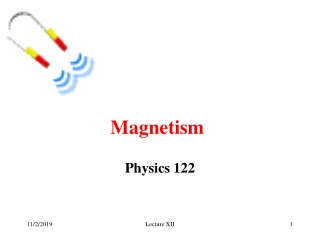DownloadDownload PresentationMagnetism

# Magnetism

Télécharger la présentation## Magnetism

- - - - - - - - - - - - - - - - - - - - - - - - - - - E N D - - - - - - - - - - - - - - - - - - - - - - - - - - -
##### Presentation Transcript

1. Magnetism Physics 122 Lecture XII

2. Magnetic force on moving charge • Magnetic force F is perpendicular to the velocity v of a particle with charge q F=qvB sin q • Magnetic field does not change the magnitude of particle’s velocity, only its direction. Charged particles move in circles in constant magnetic field Lecture XII

3. Magnetic force on moving charge • Circular motion • Magnetic force = Centripetal force F=qvB • Centripetal acceleration a=v2/R • Newton’s second law F=ma qvB=mv2/R In experiments B is known, q=e most of the time, Measure R- measure mv For a given v measure m – magnetic spectrometer Lecture XII

4. Cyclotron frequency Frequency does not depend on v! For a given particle type (q,m=const) it depends only on B Lecture XII

5. Particle selector • Crossed E and B fields: • For one given velocity electric force exactly compensates magnetic force • Only particles with this value of velocity emerge from particle selector + + + + - - - - + Lecture XII

6. Crossed fields • Electric field changes kinetic energy of charged particle qDV=mv2/2 • Magnetic field does not change KE, only direction • Lorentz force: Lecture XII

7. Torque Lecture XII

8. Current loop in magnetic field • A=ab – area of the loop • Magnetic field exerts a torque on a loop parallel to the magnetic field: Lecture XII

9. Current loop in magnetic field • q – angle between magnetic field and a perpendicular to the loop !!! • Magnetic field orients a loop current perpendicular to B. • N loops: Lecture XII

10. Magnetic moment • Current loop (area A, current I, number of turns N) in constant magnetic field B experiences torque • Define magnetic moment m perpendicular to the plane of the loop • Torque Lecture XII

11. Magnetic moment • Equilibrium position for current loop is when B is perpendicular to the face of the loop, or when magnetic moment is parallel to B • Inside magnetic field magnetic moments orient parallel to the field • Galvanometer: B is know – measure I Lecture XII

12. Magnetic field created by currents • Electric currents – moving electric charges - create magnetic field • Stationary electric charges do not create magnetic filed • First right hand rule • Thumb along the current • Wrap your fingers around the wire • fingers show the direction of the magnetic field Lecture XII

13. Magnetic field created by current • Magnetic field B created by current I at a distance r from the conductoris m0 = 4p 10-7Tm/A- magneticpermeability of free space Lecture XII

14. Ampere’s Law • In a way similar to Gauss’s law for E and Q • Not flux, integral over closed path • Only component of B parallel to dl contributes to integral Lecture XII

15. Application of Ampere’s Law • Thick wire of radius R carries current I. What is B(r ) in and outside of the wire? • Current through unit area: • Current through circle radius r<R • Path integral over radius r • Ampere’s law • For r>R – same as thin wire Lecture XII

16. Practical application: coaxial cable • Cylindrical braid carries return current  magnetic field outside is zero. Lecture XII